Most people think there is some sort of voodoo magic that goes into creating a loan amortization schedule. But I'm happy to tell you that there's not -- and even better -- even a beginner can create one easily by using Microsoft Excel.

A loan amortization table gives you a lot of good information: the total amount payable to the lender, the portion that is the interest payment, the portion that is the principal payment and the loan balance outstanding.

When a loan is amortized, it simply means an interest payment and a principal payment are combined into one periodic payment and the payment amounts are equal for each payment period. But one of the most interesting features of an amortized loan is that with each payment, the amount of interest and principal being paid changes.

## Preparing A Loan Amoritization Calculator In Excel

Excel is an extremely useful tool for calculating your very own amortization table. You can even experiment with the different inputs to see how different interest rates, repayment periods and principal loan amounts affect the loan payments you'll be making.

Let's look at an example to illustrate the process.

Assume you just purchased a car with a \$20,000 loan that has to be completely repaid in 4 years. The annual interest rate on the loan is 3%. Since the loan is amortized, the monthly payments will all be the same amount.

The first step is to calculate the monthly payment amount. This can be done using the PMT (which stands for 'payment') function in Excel.

Start by making a new spreadsheet and entering in the following data needed for the payment amount calculation.

Column A will contain the following labels for our data:

A1 = the original loan principal (your loan amount)

A2 = the loan term in years

A3 = the annual interest rate

A4 = the number of payments per year

A5 = the payment amount (what we are calculating)

Column B will contain the corresponding values for each label in column A:

B1 = \$20,000

B2 = 4

B3 = 3%

B4 = 12

B5 = the value we're looking for

Excel's PMT function can be found in the financial functions menu. First select cell B5, then click on the financial functions menu under the formulas tab and select the PMT function from the list. A box will be displayed where you should enter the data from the spreadsheet just as it is in this image:

##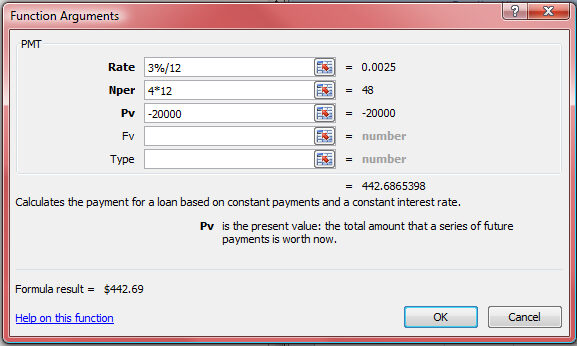A Few Things To Consider

There are a few important things to note here. First, we need to make two important adjustments, because we're calculating monthly payments. We need to divide our annual rate by 12 to get the monthly interest rate (Rate), and we need to multiply 4 by 12 to get the total number of months in our repayment period (Nper).

Second, the present value (PV) is entered as a negative value because that amount is owed to the lender.

Once your boxes are filled in, hit 'OK.'

##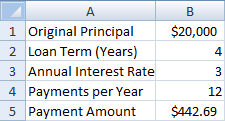Building Your Loan Amoritization Calculator

Now we will start building the amortization table.

Skip a row below the spreadsheet made in part 1, and, starting in row 7, make the following labels starting from column A and ending in column E:

A7 = Month

B7 = Payment

C7 = Interest

D7 = Principal

E7 = Balance

These are the five headings for the amortization table.

Now it is time to start filling in the table. The first step is to enter the number of months in the life of the loan. Under the 'month' heading, type '0' into cell A8 (the time the loan was issued). Fill in the subsequent cells in sequential order through number 48 (which will put you at cell A56). One trick: once you've input 0, make sure the cell is selected (A8), then drag the lower right corner of the cell down to line 56. Then select 'fill series' from the small box that appears to the right of the last cell. This should auto-populate the numeric series for the 48 months.

Next, all of the payments can be filled in because the value of each monthly payment has already been determined. Starting from month 1 (there is no payment at month 0 because payments take place at the end of each month) fill in the value \$442.69 for each month 1-48. After this, the interest and principal amounts can be calculated using their respective Excel functions.

Calculating the interest and principal components of the payments using the Excel function is simple and can be used to fill in the entire amortization table. To calculate the interest payment, select the cell C9 and then click on the financial functions menu under the formulas tab. Select the IPMT (for 'interest payment') function from the list. A box will be displayed where you should enter the data from the spreadsheet just as it is in this image:

## Notes To Know As You Work With Your Loan Amoritization Calculator

Note: The “\$” signs between the cell letters and numbers are necessary for when we copy the formulas down to the remaining cells later. The “\$” locks the referred-to cell, so the formulas stay intact when we copy them down to the remaining cells. You can lock the cells (and see the '\$') by hitting F4.

Next we can calculate the amount of principal in the payment. Similar to the IPMT calculation, select cell D9 and then click on the financial functions menu under the formulas tab. Select the PPMT (for 'principal payment) function from the list. A box will be displayed where you should enter the data from the spreadsheet just as it is in this image: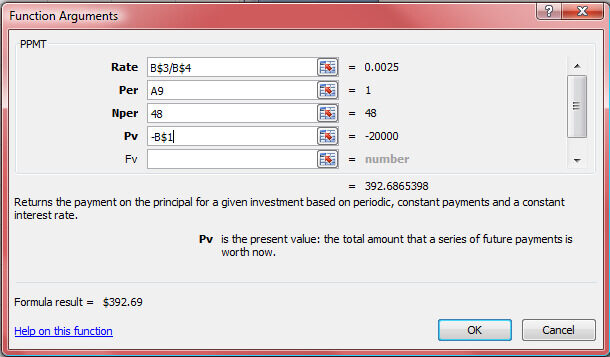Note: The principal could be calculated using this function or by simply subtracting the interest amount from the total payment amount. Likewise, the interest could be calculated if we calculated the principal first using the PPMT function and then subtracted that value from the total payment. However, it is good to know how to use both formulas, which also allows the rest of the table to be filled out more efficiently as you will see below.

The final column (Column E) is the remaining balance column. This can be easily calculated for each period now that we have determined the amounts of interest and principal. For month 0, no payments have been made yet, so the balance is \$20,000. However, we want to command Excel to automatically calculate the balance in each period, so instead of manually entering “\$20,000,” enter “=B1,” referring to the above table for payment calculation. For month 1, since the balance is calculated by subtracting the previous month’s balance by the current month’s principal payment, enter “=E8-D9” into cell E9. The balance will automatically be calculated. Your amortization table is now complete for month 1 and should look exactly like this: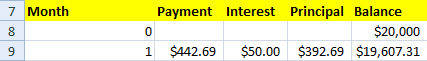All the data for the remaining months can be filled in by simply selecting the cells B9-E9, copying the selection, and then pasting the selecting into cells B10-E10. Since we have entered the formulas for each cell and locked our cells that will be used in every calculation, the values for interest, principal and balance are automatically calculated. Seeing that work for month 2, the entire table can be completed by simply selecting columns B-E through month 48 and pasting the already copied information. Excel has now calculated every value for the amortization table using the formulas we entered. Your completed table should look just like this:

First Year of Loan: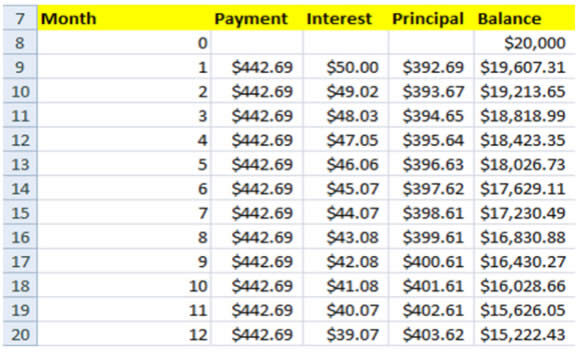Last Year of Loan: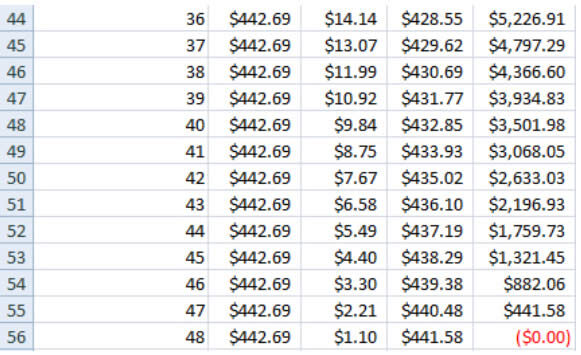Note: These tables contain only the first and last years of the amortization table. Years 2 and 3 would be between these two tables forming one continuous amortization table for all 48 months.

If you're interested in calculating mortgage payments for a fixed-rate mortgage, you can make an amortization table yourself using these steps, or you can use our financial calculator to do it for you. Our mortgage calculator will show you your monthly payment as well as a full amortization schedule.